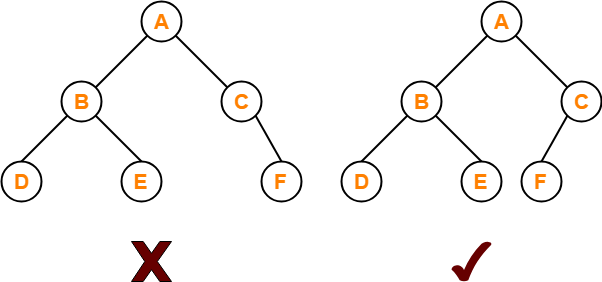New update is available. Click here to update.

# Count Complete Binary Tree Nodes

Last Updated: 20 Dec, 2020
Difficulty: Easy

## PROBLEM STATEMENT

#### In a complete binary tree every level, except possibly the last, is completely filled, and all nodes in the last level are as far left as possible.

##### For example:
``````For the binary trees in the image below.
````````````The left tree in the image is not a complete binary tree that’s why it is invalid and the right tree in the image is a valid complete binary tree which contains total 6 nodes.
``````
##### Input Format:
``````The first line contains an integer 'T' which denotes the number of test cases or queries to be run. Then the test cases are as follows.

The only line of each test case contains elements in the level order form. The line consists of values of nodes separated by a single space. In case a node is null, we take -1 in its place. So -1 would not be a part of the tree nodes.

For example, the input for the tree depicted in the below image will be:
````````````For example taking a tree:

1
2 3
4 -1 5 6
-1 7 -1 -1 -1 -1
-1 -1
``````

#### Explanation :

``````Level 1 :
The root node of the tree is 1

Level 2 :
Left child of 1 = 2
Right child of 1 = 3

Level 3 :
Left child of 2 = 4
Right child of 2 = null (-1)
Left child of 3 = 5
Right child of 3 = 6

Level 4 :
Left child of 4 = null (-1)
Right child of 4 = 7
Left child of 5 = null (-1)
Right child of 5 = null (-1)
Left child of 6 = null (-1)
Right child of 6 = null (-1)

Level 5 :
Left child of 7 = null (-1)
Right child of 7 = null (-1)

The first not-null node(of the previous level) is treated as the parent of the first two nodes of the current level. The second not-null node (of the previous level) is treated as the parent node for the next two nodes of the current level and so on.

The input ends when all nodes at the last level are null(-1).
``````
##### Note :
``````The above format was just to provide clarity on how the input is formed for a given tree.
The sequence will be put together in a single line separated by a single space. Hence, for the above-depicted tree, the input will be given as:

1 2 3 4 -1 5 6 -1 7 -1 -1 -1 -1 -1 -1
``````
##### Output Format:
``````For each test case, print the number of nodes in the given binary tree.

Print the output of each test case in a separate line.
``````
##### Note:
``````You don’t need to print anything, it has already been taken care of.
``````
##### Constraints:
``````1 <= T <= 100
0 <= N <= 3000
0 <= val <= 10^5

Where ‘T’ is the number of test cases, and ‘N’ is the total number of nodes in the binary tree, and “val” is the value of the binary tree node

Time Limit: 1 sec
``````## Approach 1

Our intuition is to calculate the number of nodes step by step from the leaf nodes. For that, we will use a recursive approach where we keep track of both the number of nodes in left and right subtrees.

For each subtree the total number of nodes will be :

``1 + countNodes(root->left) + countNodes(root->right)``